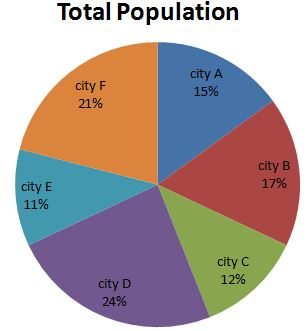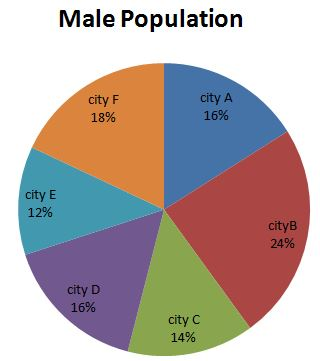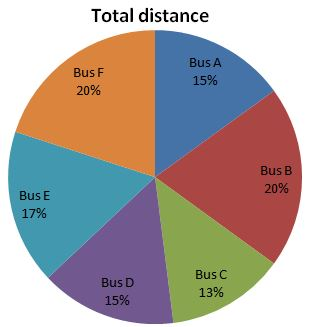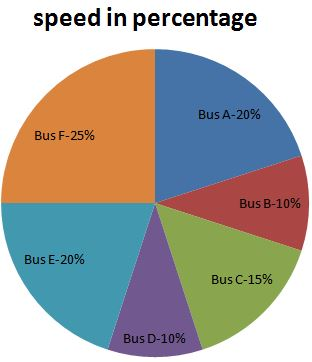#### D.1-5) Go through the data set given below and solve the questions based on it. The pie chart 1 shows the percentage of people in different cities. The pie chart shows the percentage of male in different cities.1) In city D total males are 256, what is the total number of females in city A and F?

a) 2088

b) 1988

c) 1978

d) 1878

e) None of these

(B)

In city D male percentage 16%= 256.

“Then, total people in city D =”  “256” /”16″  “×100= 1600”

Total percentage of people in city D, 24% = 1600

“Then, total people in city A =”  “1600” /”24″  “×15″=1000

Male percentage in city A 16% then female percentage is 84%

Total female in city A = 84% of 1000= 840

“Then, total people in city F =”  “1600” /”24″  “×21″=1400

Male percentage in city F 18% then female percentage is 82%

Total female in city F = 82% of 1400= 1148

Required total = 1148+840 = 1988

2) In city E females are 1936, What is the ratio of males in city C and city D?

a) 7:11

b) 16:7

c) 11:7

d) 7:16

e) None of these

(D)

In city E female percentage is 88%

“Then total people in city E =”  “1936” /”88″  “×100=2200”

Total percentage of people in city E, 11% = 2200

“Then total people in city C =”  “2200” /”11″  “×12″=2400

Male percentage in city C 14% then female percentage is 86%

Total males in city C = 14% of 2400= 336

Total percentage of people in city E, 11% = 2200

“Then total people in city D =”  “2200” /”11″  “×24 = 4800”

Male percentage in city D 16% then female percentage is 84%

Total males in city D = 16% of 4800= 768

Ratio of males in city C and city D = 336:768 = 7:16.

3) If Ratio between male people in city B and E is 24:13. The different between the male people in city B and E is 132, what is the difference between the female people in city B and city E?

a) 242

b) 238

c) 234

d) 232

e) 221

(D)

The Ratio between male people in city B and E is 24x:13x

The difference between the male people in city B and E is 132

Then, 11x = 132

x = 12

Hence, male people in city B = 24× 12 =288 is 24% of the total people in city B

“then female people in city B =”  “288” /”24″  “×76 = 912 “

Male people in city E = 13 ×12 = 156 is 12% of the total people in city E,

“then female people in city E  =”  “156” /”12″  “×88= 1144 “

Required difference = 1144- 912 = 232

4) In city A’s total population is 3000, find the total number of female in city A, B, C, D, E, F?

a) 16576

b) 16850

c) 158680

d) 162580

e) None of these

(A)

In city A total population =3000

Female people in city A = 84% of 3000 = 2520

In city B total population =3000/15×17 =3400

Female people in city B = 76% of 3400 = 2584

In city C total population =3000/15×12 =2400

Female people in city C = 86% of2400 = 2064

In city D total population =3000/15×24 =4800

Female people in city D = 84% of 4800 = 4032

In city E total population =3000/15×11 =2200

Female people in city E = 88% of 2200 = 1936

In city F total population =3000/15×21 =4200

Female people in city F = 82% of4200 = 3440

Required total = 16576

5) From the data obtained in the question (Q.64). Find the total number of Male people in all cities together?

a) 3784

b) 3864

c) 3584

d) 3424

e) None of these

(D)

Total number of males= 480+ 816 + 336 + 768 +264 + 760 = 3424

#### D.6-10) Go through the data set given below and solve the questions based on it. The pie chart 1 shows percentage of distance of various buses. The pie chart 2 shows percentage of the speed of various buses in km/hr.Total Speed = 120 KMPH6) The total time taken by bus F is 2hr, how much time taken by bus C and Bus A?

a) 4.035 hr

b) 6 hr

c) 5 hr

d) 8 hr

e) Cannot be determined

(A)

Bus F speed = 120×25/100=30 km/hr.

Distance = speed × time = 30× 2 = 60km.

Bus F distance 20% = 60 km

Bus C distance =60/20×13= 39 km

Bus C  time =39/18= 2.16 hr

Similarly,

Bus A distance =60/20×15 =45 km

Bus A  time =45/24= 1.875 hr

Total time =2.16+1.875=4.035hr

7) If bus C distance is 78km then what is the difference between the time taken by bus D and A?

a) 1.2 hr

b) 3.8 hr

c) 4 hr

d) 3.75 hr minutes

e) None of these

(D)

Bus C distance 13% = 78 km

Bus A distance =78/13×15 = 90 km

speed of A=120×20/100=24 kmph

Then Bus A time =90/24= 3.75hr

Similarly,

Bus D distance =78/13×15 = 90 km

speed of D=120×10/100=12 kmph

Then Bus D time =90/12= 7.5hr

Required time difference = 7.5 – 3.75 = 3.75 hr

8) The difference between the distance of bus D and E is 40km, How many more hour taken by bus D than Bus E?

a) 7 hour 56 minutes

b) 6 hour 35 minutes

c) 10 hour 50 minutes

d) 5.5 hour 40 minutes

e) Cannot be determined

(C)

Difference between bus D and bus E is 2% (i.e., 2% = 40)

Bus D distance =40/2×15= 300 km

speed of D=120×10/100=12 kmph

Bus D Time =300/12=25 hr

Similarly,

Bus E distance =40/2×17= 340 km

speed of E=120×20/100=24 kmph

Bus E Time =340/24=14.16 hr

Hence, Bus D has taken 10 hour 50 minutes more than Bus E.

9) If the bus B distance is 400km, what is the total time taken by bus C, A, D, E?

a) 66 hour 3 min

b) 72 hour 5 min

c) 75 hour

d) 85 hour 6 min

e) None of these

(E)

Bus B distance 20% = 400km

Bus C distance =400/20×13= 260 km

Speed of C=120×15/100=18 hr

Bus C time =260/18=14.44 hour

Bus A distance =400/20×15= 300 km

Speed of A=120×20/100=24 kmph

Bus A time =300/24=12.5 hour

Bus D distance =400/20×15= 300 km

Speed of D=120×10/100=12kmph

Bus D time =300/12=25 hour

Bus E distance =400/20×17= 340 km

Speed of E=120×20/100=24 kmph

Bus E time =340/24=14.16 hour

Total time =(14.44+12.5+25+14.16)=66hour 6 minutes

10) If the bus F’s time taken is 2 hr, how much time taken by Bus B?

a) 4.8 hr

b) 3.5 hr

c) 5 hr

d) 4.5 hr

e) None of these

(D)

Bus F speed = 120×25/100=30 km/hr.

Bus F distance = speed × time = 30 × 2 = 60km.

Bus F distance 20% = 60 km

Bus B distance =60/20×20 = 60 km

speed of Bus B=120×10/100=12kmph

Bus B  time =60/12= 5hr